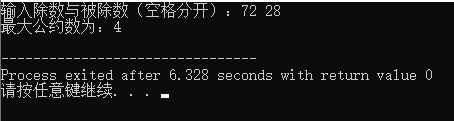• 辗转相除法最大公约数的问题C语言版求解，供大家学习C/C++
• 辗转相除法  「辗转相除法」又叫做「欧几里得算法」,是公元前 300 年左右的希腊数学家欧几里得在他的著作《几何原本》提出的.利用这个方法,可以较快地求出两个自然数的最大公因数,即 HCF 或叫做 gcd....
辗转相除法 「辗转相除法」又叫做「欧几里得算法」,是公元前 300 年左右的希腊数学家欧几里得在他的著作《几何原本》提出的.利用这个方法,可以较快地求出两个自然数的最大公因数,即 HCF 或叫做 gcd.最大公约数（greatest common divisor，简写为gcd；或highest common factor，简写为hcf)所谓最大公因数,是指几个数的共有的因数之中最大的一个,例如 8 和 12 的最大公因数是 4,记作 gcd(8,12)=4. 在介绍这个方法之前,先说明整除性的一些特点,注以下文的所有数都是正整数,以后不再重覆. 我们可以这样给出整除以的定义: 对於两个自然数 a 和 b,若存在正整数 q,使得 a=bq,则 b 能整除 a,记作 b | a,我们叫 b 是 a 的因数,而 a 是 b 的倍数. 那麼如果 c | a,而且 c | b,则 c 是 a 和 b 的公因数. 由此,我们可以得出以下一些推论: 推论一:如果 a | b,若 k 是整数,则 a | kb.因为由 a | b 可知 ha=b,所以 (hk)a=kb,即 a | kb. 推论二:如果 a | b 以及 a | c,则 a | (b±c).因为由 a | b 以及 a | c,可知 ha=b,ka=c,二式相加,得 (h+k)a=b+c,即 a | (b+c).同样把二式相减可得 a | (b-c). 推论三:如果 a | b 以及 b | a,则 a=b.因为由 a | b 以及 b | a,可知 ha=b,a=kb,因此 a=k(ha),hk=1,由於 h 和 k 都是正整数,故 h=k=1,因此 a=b. 辗转相除法是用来计算两个数的最大公因数,在数值很大时尤其有用而且应用在电脑程式上也十分简单.其理论如下: 如果 q 和 r 是 m 除以 n 的商及余数,即 m=nq+r,则 gcd(m,n)=gcd(n,r). 证明是这样的: 设 a=gcd(m,n),b=gcd(n,r) 则有 a | m 及 a | n,因此 a | (m-nq)(这是由推论一及推论二得出的),即 a | r 及 a | n,所以 a | b 又 b | r 及 b | n,所以 b | (nq+r),即 b | m 及 b | n,所以b | a.因为 a | b 并且 b | a,所以 a=b,即 gcd(m,n)=gcd(n,r). 例如计算 gcd(546, 429),由於 546=1(429)+117,429=3(117)+78,117=1(78)+39,78=2(39),因此 gcd(546, 429) =gcd(429, 117) =gcd(117, 78) =gcd(78, 39) =39
public class GcdTest
{
//循环实现
int gcd1(int a, int b)
{
int k = 0;
do
{
//得到余数
k = a % b;
//根据辗转相除法,把被除数赋给除数
a = b;
//余数赋给被除数
b = k;
} while (k != 0);
//返回被除数
return a;
}
//递归实现
int gcd2(int a,int b)
{
//直到满足此条件逆归退出
if(b == 0)
{
return a;
}
if(a < 0)
{
return gcd2(-a,b);
}
if(b < 0)
{
return gcd2(a,-b);
}
return gcd2(b,a % b);
}

public static void main(String[] args)
{
GcdTest gt = new GcdTest();
System.out.println(gt.gcd1(12,24));
System.out.println(gt.gcd2(12,24));
}

}


展开全文c string class 算法
• 对于辗转相除法的证明：https://blog.csdn.net/er111er/article/details/79251895 原作者写得很棒。 def gcd(a,b): return a if b==0 else gcd(b,a%b) 首先核心在于：a和b的最大公约数等价于b和a对b求余的最大公...
对于辗转相除法的证明：https://blog.csdn.net/er111er/article/details/79251895 原作者写得很棒。
	def gcd(a,b):
return a if b==0 else gcd(b,a%b)

首先核心在于：a和b的最大公约数等价于b和a对b求余的最大公约数。 感性理解，假设存在最大公约数M，那么a是M的倍数，b是M的倍数，那么a和b的线性组合也是M的倍数。 所以a对b的余数是线性组合的一种，那么也是M的倍数，更重要的是a对b的余数小于a和b（a>b的时候)，这样就使得问题可以递归求解。
展开全文数学
• 辗转相除法，一种求最大公约数的算法已知：A / B = C ······ R (A、B、C、R皆是整数)假设：D是A的余数，D也是B的余数，那么D就是A和B的公约数D是A和B的约数，则A和B是D的倍数，B * C也是D的倍数既然A与B*C都是...

辗转相除法，一种求最大公约数的算法
已知：A / B = C ······ R  (A、B、C、R皆是整数)
假设：D是A的余数，D也是B的余数，那么D就是A和B的公约数
D是A和B的约数，则A和B是D的倍数，B * C也是D的倍数
既然A与B*C都是D的倍数，那么A与B*C的差也是D的倍数
A - B*C = R
所以R也是D的倍数
如果D是A或B的公约数，那么D也是B和R的公约数
故：(A,B)= (B,R)
由以上证明则可以求出最大的公约数
例如：求72和28的最大公约数
72 / 28 = 2 ······ 16
↓      ↓      ↓          ↓
28 / 16 = 1 ······ 12
↓      ↓      ↓          ↓
16 / 12 = 1 ······ 4
↓      ↓      ↓         ↓
12 / 4  =  3  ······ 0
现在可以知道 72与28的最大公约数是4
1 #include
2 int main(){
3 int a; // 除数
4 int b; // 被除数
5 int r=1; 　// 余数,赋初值为1
6 printf("输入除数与被除数(空格分开)：");
7 scanf("%d %d",&a,&b);
8 while(r!=0){ // 如果a
9 r = a % b;
10 a = b;
11 b = r;
12 }
13 printf("最大公约数为：%d\n",a); // 此时b的值已经在a中了，所以输出的a就是最大公约数
14 return 0;
15 }展开全文• ## 求最大公约数（辗转相除法）

万次阅读 多人点赞 2019-06-03 16:20:50
最大公约数（Greatest Common Divisor）指两个或多个整数共有约数中最大的...求最大公约数有多种 方法，常见的有质因数分解法、短除法、辗转相除法、更相减损法。与最大公约数相对应的概念是最小公倍数，a，b的 最小...

最大公约数（Greatest Common Divisor）指两个或多个整数共有约数中最大的一个。
也称最大公因数、最大公因子，a， b的最大公约数记为（a，b），同样的，a，b，c的最大 公约数记为（a，b，c），多个 整数的最大公约数也有同样的记号。求最大公约数有多种 方法，常见的有 质因数分解法、 短除法、 辗转相除法、 更相减损法。与最大公约数相对应的概念是 最小公倍数，a，b的 最小公倍数记为[a，b]。

再来介绍一下辗转相除法：

辗转相除法又叫欧几里得算法,是欧几里得最先提出来的.辗转相除法的实现,是基于下面的原理(在这里用(a,b)表示a和b的最大公因数)： 　　(a,b)=(a,ka+b),其中a、b、k都为自然数.………………① 　　也就是说,两个数的最大公约数,将其中一个数加到另一个数上,得到的新数,其公约数不变,比如(4,6)=(4+6,6)=(4,6+2×4)=2.要证明这个原理很容易：如果p是a和ka+b的公约数,p整除a,也能整除ka+b.那么就必定要整除b,所以p又是a和b的公约数,从而证明他们的最大公约数也是相等的. 　　基于上面的原理,就能实现我们的迭代相减法： 　　(78,14)=(64,14)=(50,14)=(36,14)=(22,14)=(8,14)=(8,6)=(2,6)=(2,4)=(2,2)=(0,2)=2 　　基本上思路就是大数减去小数,一直减到能算出来为止,在作为练习的时候,往往进行到某一步就已经可以看出得值.迭代相减法简单,不过步数比较多,实际上我们可以看到,在上面的过程中,由(78,14)到(8,14)完全可以一步到位,因为(78,14)=(14×5+8,14)=(8,14),由此就诞生出我们的辗转相除法. 　　用辗转相除法求(a,b).设r0=b,r1=a,反复运用除法算式,得到一系列整数qi,ri和下面的方程： 　　相当于每一步都运用原理①把数字进行缩小,上面右边就是每一步对应的缩小结果,可以看出,最后的余数rn就是a和b的公约数.迭代相减法和辗转相除法在本质上是一样的,相对来说,减法比较简单（需要10步）,但是除法步数少（仅需4步）.

因此可以通过这个原理来求出最大公约数：
#include<iostream>
#include<cstdio>
using namespace std;

int fun(int m,int n){
int rem;			//余数，当余数为0的时候，最后的m即为最大公约数
//先用较小的数对较大的数取余，再用余数对较小的数求余，直到余数为零
while(n > 0){
rem = m % n;
m = n;
n = rem;
}
return m;			//将结果返回
}
int main(){
int n,m;
cin>>m>>n;
cout<<"m和n的最大公约数为："<<fun(m,n)<<endl;
return 0;
} 
因为到余数为零结束，所以还可以将程序简化一下用递归来求：
int fun(int m,int n){
if(n==0) return m;
return fun(n,m%n);
}
再简化一下，用一行代码来求：
int gcd(int m, int n) {
return n ? gcd(n, m % n) : m;
}

展开全文• 主要介绍了Python基于辗转相除法求解最大公约数的方法,结合实例形式分析了Python使用辗转相除法求解最大公约数的实现方法与优化操作技巧,需要的朋友可以参考下
• 主要介绍了Java中使用辗转相除法最大公约数,本文直接给出代码实例,需要的朋友可以参考下
• m = 9147485 n = 5147480 辗转相除法最大公约数 最大公约数=？
• 辗转相除法求两个数的最大公约数（Greatest common divisor，GCD），在公元前300年前由欧几里得发现，被称为世界上最古老的算法。
• 辗转相除法辗转相除法是求两个自然数的最大公约数的一种方法，也叫欧几里德算法。 例如，求（319，377）： ∵ 319÷377=0（余319） ∴（319，377）=（377，319）； ∵ 377÷319=1（余58） ∴（377，319）=...
• 辗转相除法最大公约数（Python） #辗转相除法 #1大数除以小数得到余数 #2用余数除以1中的除数得到余数 #3重复2直到余数等于0返回除数即为两数的最大公约数 def eucAlg(a,b): if a<b: c=a a=b b=c while 1:...Python
• ## c语言 辗转相除法求最大公约数

万次阅读 多人点赞 2016-09-22 21:34:22c语言
•Java 算法
• Python辗转相除求最大公约数 ...输入2个数，用辗转相除法最大公约数 def gcd(x,y): if x < y: x,y = y,x while (x % y) != 0: r = x % y x = y y = r return y while True: try: a,b...
• ## C语言的辗转相除法求最大公约数

万次阅读 多人点赞 2019-04-04 07:45:54
在数学中，辗转相除法，又称欧几里得算法（英语：Euclidean algorithm），是求最大公约数的算法。辗转相除法首次出现于欧几里得的《几何原本》（第VII卷，命题i和ii）中，而在中国则可以追溯至东汉出现的《九章算术...
• 辗转相除法最大公因数 辗转相除法是求最大公因数的一种方法，其原理和更相减损术有异曲同工之处，所以可以由更相减损术的原理引入辗转相除法的原理。 更相减损术的原理 假设现有两个数161和63，要求这两个数的最大...
• 辗转相除法最大公约数证明 假设整数aaa，bbb，且a>ba > ba>b，rrr为aaa除以bbb的余数，则有(a,b)=(b,c)。 证明： 假设d=(a,b)d=(a,b)d=(a,b)，则d∣a,d∣b⇒d∣a−k∗b(k≥0)⇒d∣rd|a,d|b \Rightarrow d...数学
• 辗转相除法辗转相除法」又叫做「欧几里得算法」,是公元前 300 年左右的希腊数学家欧几里得在他的著作《几何原本》提出的.利用这个方法,可以较快地求出两个自然数的最大公因数,即 HCF 或叫做 gcd.所谓最大公因数,是...
• 利用辗转相除法求两个数的最大公约数。要求能接受用户的输入，输出最大公约数辗转相除法原理： 已知a,b,c为正整数，若a除以b余c，则（a,b）=(b,c)，其中用（a,b）来表示a和b的最大公约数。（证明过程请参考其它......